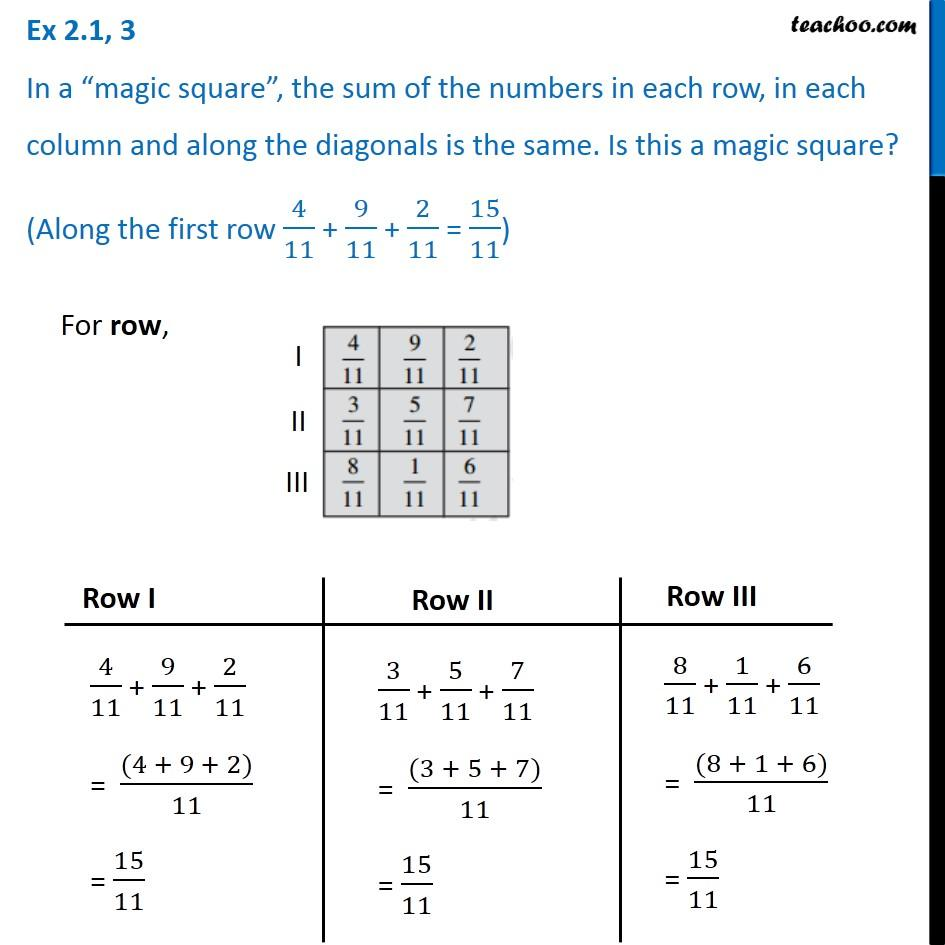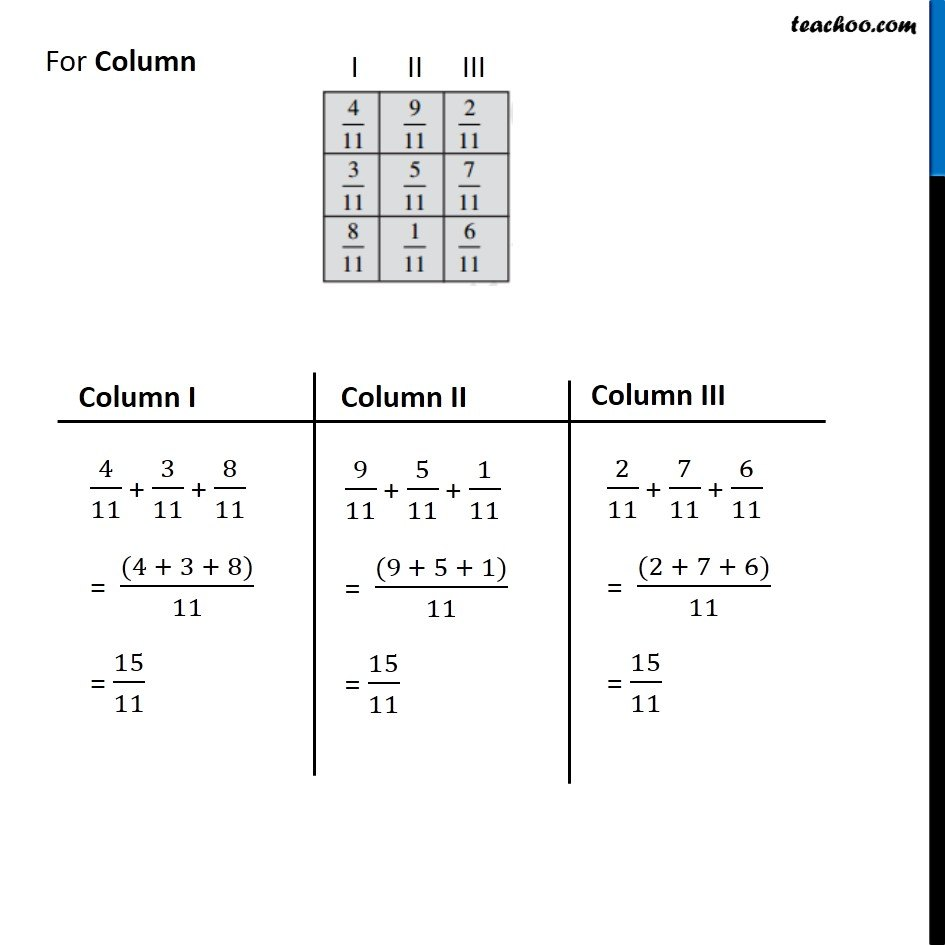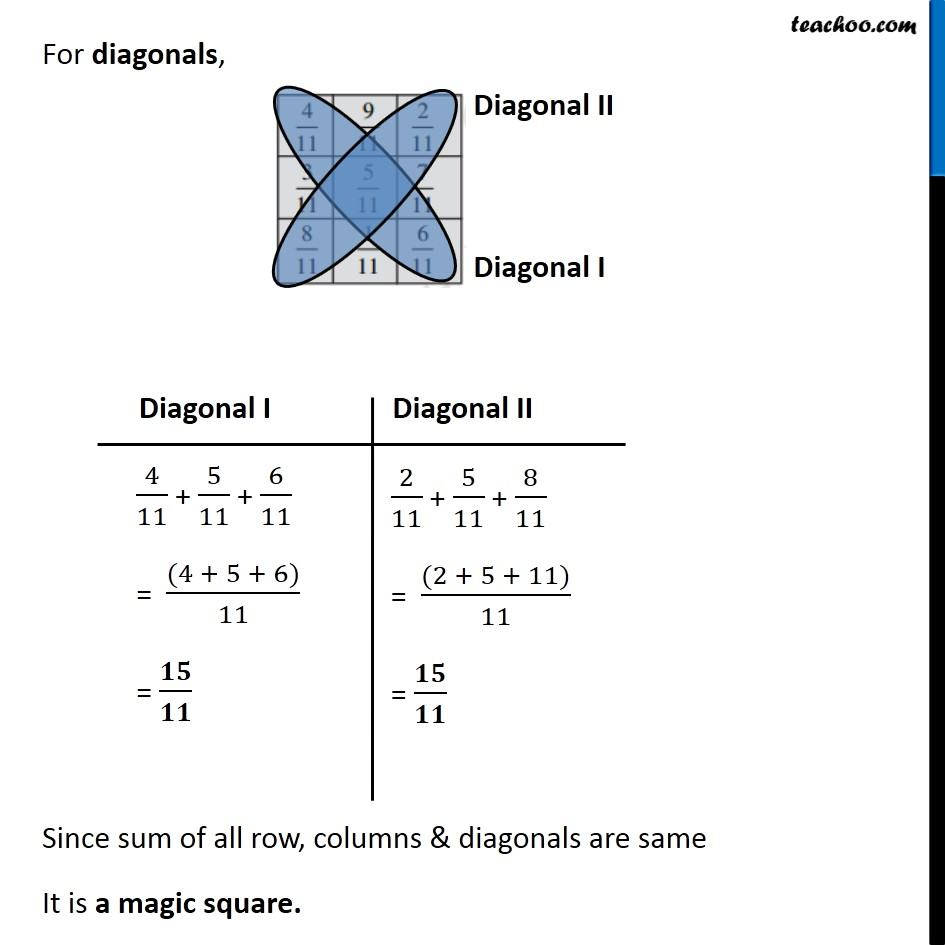Subscribe to our Youtube Channel - https://you.tube/teachoo

1. Chapter 2 Class 7 Fractions and Decimals
2. Concept wise
3. Addition and Subtraction of Fractions

Transcript

Ex 2.1, 3 In a “magic square”, the sum of the numbers in each row, in each column and along the diagonals is the same. Is this a magic square? (Along the first row 4/11 + 9/11 + 2/11 = 15/11) 4/11 + 9/11 + 2/11 = ((4 + 9 + 2))/11 = 15/11 3/11 + 5/11 + 7/11 = ((3 + 5 + 7))/11 = 15/11 8/11 + 1/11 + 6/11 = ((8 + 1 + 6))/11 = 15/11 4/11 + 3/11 + 8/11 = ((4 + 3 + 8))/11 = 15/11 9/11 + 5/11 + 1/11 = ((9 + 5 + 1))/11 = 15/11 For diagonals, Since sum of all row, columns & diagonals are same It is a magic square. 4/11 + 5/11 + 6/11 = ((4 + 5 + 6))/11 = 𝟏𝟓/𝟏𝟏 2/11 + 5/11 + 8/11 = ((2 + 5 + 11))/11 = 𝟏𝟓/𝟏𝟏